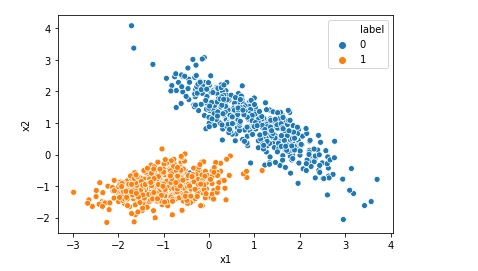# Implementing Logistic Regression in 10 lines in Python

Logistic Regression is one of the most popular Machine Learning algorithm used for the classification problems. It should be noted that though there is a regression word in the name of the algorithm Logistic Regression, it is used for classification problems.

A use case of Logistic regression could be, based on the symptoms for a disease that a patient has Logistic regression may be used to predict the risk of developing a given disease like cancer.

Another application could be to predict whether a customer will buy a product or not based on customer buying history.

In this article we are going to implement a logistic regression model using python and sklearn, where data has two features x1 and x2 and one traget variable y having two classes, 0 and 1. So this is a binary classification problem.

If you like to check out Logistic Regression in Python from Scratch please watch video:

Ok so lets start the coding part and Import the necessary libraries.

``````import pandas as pd
from sklearn import metrics
from sklearn.linear_model import LogisticRegression
from sklearn.model_selection import train_test_split
import seaborn as sns``````

After that we can load the data in pandas data frame.

``````df = pd.read_csv('Classification-Data.csv')

As you can see in above image, we have two features, named x1 and x2 in the data and there is one binary label column with values either 0 or 1.

Now we need to split the data into train and test part as below.

``x_train, x_test, y_train, y_test = train_test_split(x, y, random_state=42)``

Then we need to define the model and assign it to a variable log-reg.

``log_reg = LogisticRegression() ``

After that we will train the model using fit method.

``log_reg.fit(x_train, y_train)``

Its time to predict the values now as the model is trained.

``y_pred = log_reg.predict(x_test) ``

Finally we are going to evaluate the model as below

``accuracy = metrics.accuracy_score(y_test, y_pred)``

End Notes

In this model we learned how to implement a Logistic Regression model in Python. We had two feature and two classes. We used sklearn for the implementation. You can find data and code here.

Logistic Regression has many other important concepts and coding like

Confusion Matrix

Classification Report

Receiver Operating Characteristic Area Under Curve

You may like to explore above posts.

Happy Coding !!